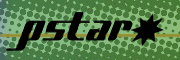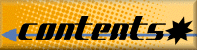### Appendix: Significance of Calculated Quantities

Collision stopping power: average rate of energy loss per unit path length, due to Coulomb collisions that result in the ionization and excitation of atoms. For heavy charged particles, the collision stopping power is often called electronic stopping power.

Density-effect correction: enters into the formula for the collision stopping power and takes into account the reduction of the collision stopping power due to the polarization of the medium by the incident electron.

Radiative stopping power: average rate of energy loss per unit path length due to collisions with atoms and atomic electrons in which bremsstrahlung quanta are emitted. Important only for electrons.

Nuclear stopping power: average rate of energy loss per unit path length due to the transfer of energy to recoiling atoms in elastic collisions. Important only for heavy charged particles.

Total stopping power: for electrons, the sum of the collision and radiative stopping powers; for protons and helium ions, the sum of collision and nuclear stopping powers.

CSDA range: a very close approximation to the average path length traveled by a charged particle as it slows down to rest, calculated in the continuous-slowing-down approximation. In this approximation, the rate of energy loss at every point along the track is assumed to be equal to the total stopping power. Energy-loss fluctuations are neglected. The CSDA range is obtained by integrating the reciprocal of the total stopping power with respect to energy.

Projected range: average value of the depth to which a charged particle will penetrate in the course of slowing down to rest. This depth is measured along the initial direction of the particle.

Detour factor: ratio of the projected range to the CSDA range. As the result of multiple scattering, the trajectory of the particle is wiggly rather than straight, and the detour factor is always smaller than unity.

Radiation yield: average fraction of the initial kinetic energy of an electron that is converted to bremsstrahlung energy as a particle slows down to rest, calculated in the continuous-slowing-down approximation. Important only for electrons.

Introduction   |   ESTAR   |   PSTAR and ASTAR   |   References   |   Appendix